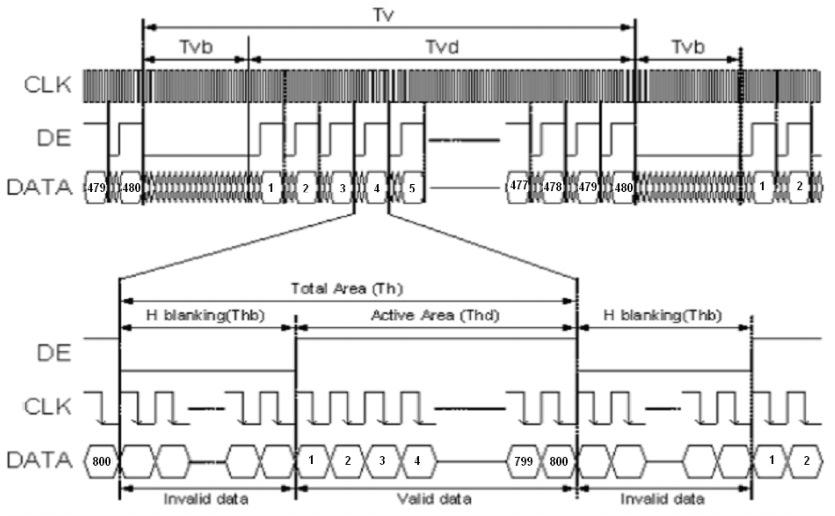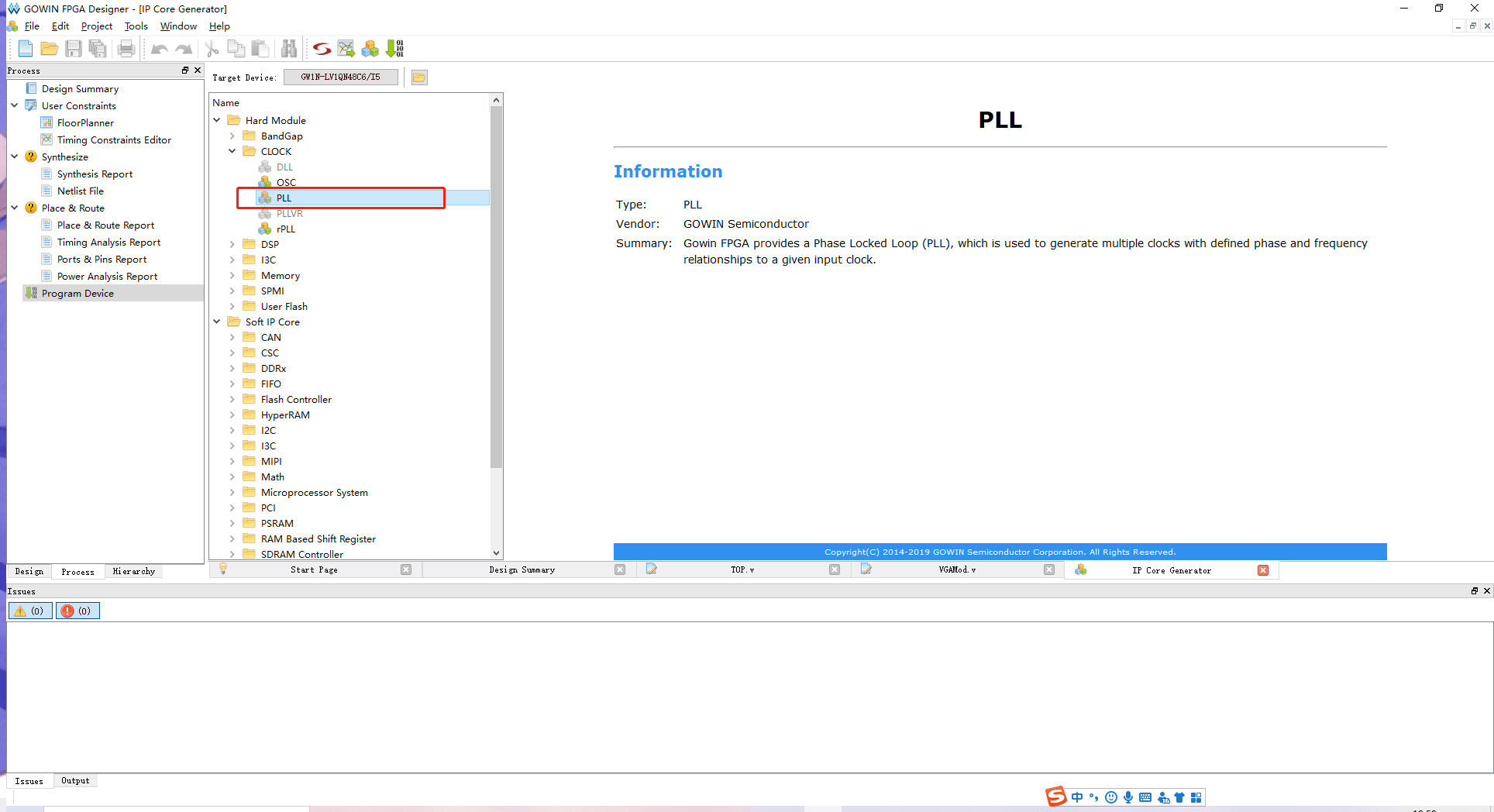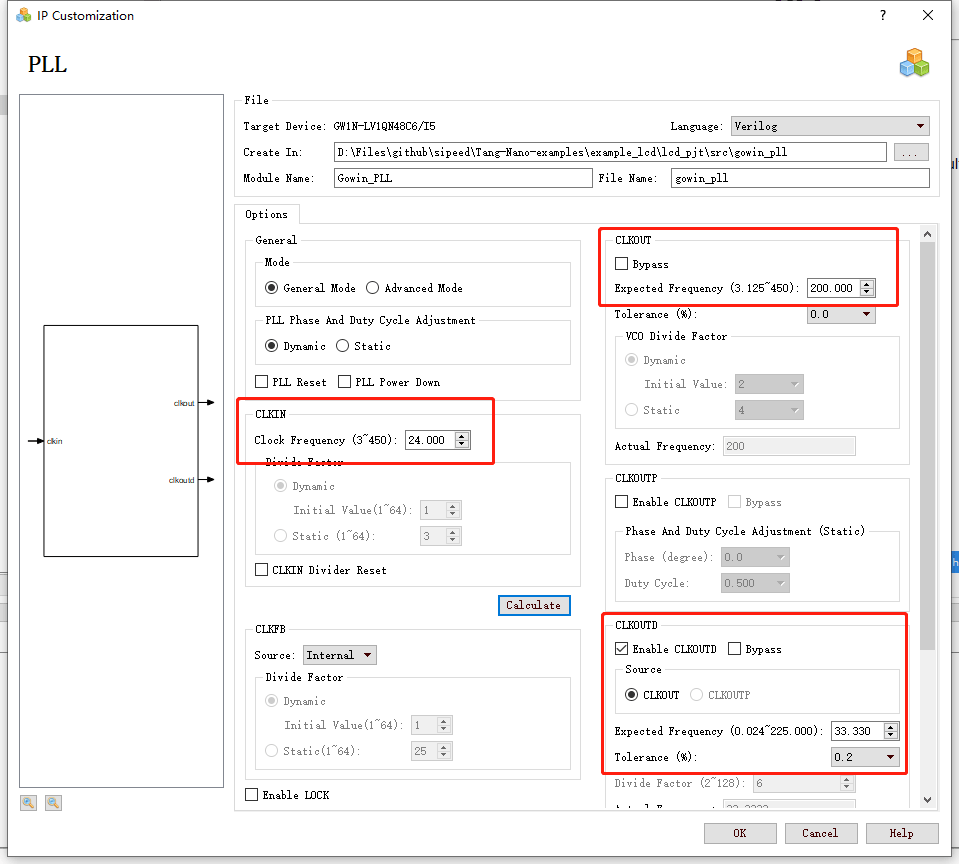RGB LCD 示例

# 先介绍时序

RGB LCD 显示图像的原理和 VGA 类似，都是在计算机内部以数字的方式生成需要显示的图像信息，再通过模数转换的方式，将这些数字的图像信息转变为 RGB 三原色模拟信号，以及行、场同步信号。# Verilog实现

## pll## lcd时序产生

``````localparam      V_BackPorch = 16'd6; //0 or 45
localparam      V_Pluse     = 16'd5;
localparam      HightPixel  = 16'd480;
localparam      V_FrontPorch= 16'd62; //45 or 0

localparam      H_BackPorch = 16'd182;  //NOTE: 高像素时钟时，增加这里的延迟，方便K210加入中断
localparam      H_Pluse     = 16'd1;
localparam      WidthPixel  = 16'd800;
localparam      H_FrontPorch= 16'd210;

localparam      PixelForHS  =   WidthPixel + H_BackPorch + H_FrontPorch;
localparam      LineForVS   =   HightPixel + V_BackPorch + V_FrontPorch;
``````

``````always @(  posedge PixelClk or negedge nRST  )begin
if( !nRST ) begin
LineCount       <=  16'b0;
PixelCount      <=  16'b0;
end
else if(  PixelCount  ==  PixelForHS ) begin
PixelCount      <=  16'b0;
LineCount       <=  LineCount + 1'b1;
end
else if(  LineCount  == LineForVS  ) begin
LineCount       <=  16'b0;
PixelCount      <=  16'b0;
end
end

//注意这里HSYNC和VSYNC负极性
assign  LCD_HSYNC = (( PixelCount >= H_Pluse)&&( PixelCount <= (PixelForHS-H_FrontPorch))) ? 1'b0 : 1'b1;
assign  LCD_VSYNC = ((( LineCount  >= V_Pluse )&&( LineCount  <= (LineForVS-0) )) ) ? 1'b0 : 1'b1;
``````

``````assign  LCD_DE = (  ( PixelCount >= H_BackPorch )&&
( PixelCount <= PixelForHS-H_FrontPorch ) &&
( LineCount >= V_BackPorch ) &&
( LineCount <= LineForVS-V_FrontPorch-1 ))  ? 1'b1 : 1'b0;
//这里不减一，会抖动
``````

``````assign  LCD_R   =   (PixelCount<200)? 5'b00000 :
(PixelCount<240 ? 5'b00001 :
(PixelCount<280 ? 5'b00010 :
(PixelCount<320 ? 5'b00100 :
(PixelCount<360 ? 5'b01000 :
(PixelCount<400 ? 5'b10000 :  5'b00000 )))));

assign  LCD_G   =   (PixelCount<400)? 6'b000000 :
(PixelCount<440 ? 6'b000001 :
(PixelCount<480 ? 6'b000010 :
(PixelCount<520 ? 6'b000100 :
(PixelCount<560 ? 6'b001000 :
(PixelCount<600 ? 6'b010000 :
(PixelCount<640 ? 6'b100000 : 6'b000000 ))))));

assign  LCD_B   =   (PixelCount<640)? 5'b00000 :
(PixelCount<680 ? 5'b00001 :
(PixelCount<720 ? 5'b00010 :
(PixelCount<760 ? 5'b00100 :
(PixelCount<800 ? 5'b01000 :
(PixelCount<840 ? 5'b10000 :  5'b00000 )))));
``````

``````VGAMod  D1
(
.CLK        (   CLK_SYS     ),
.nRST       (   nRST        ),

.PixelClk   (   CLK_PIX     ),
.LCD_DE     (   LCD_DEN     ),
.LCD_HSYNC  (   LCD_HYNC    ),
.LCD_VSYNC  (   LCD_SYNC    ),

.LCD_B      (   LCD_B       ),
.LCD_G      (   LCD_G       ),
.LCD_R      (   LCD_R       )
);
``````